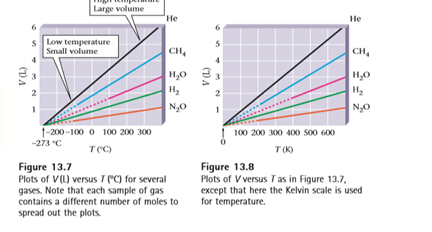Chapter 13, Problem 26QAP### Introductory Chemistry: A Foundati...

9th Edition
Steven S. Zumdahl + 1 other
ISBN: 9781337399425

#### Solutions

Chapter
Section### Introductory Chemistry: A Foundati...

9th Edition
Steven S. Zumdahl + 1 other
ISBN: 9781337399425
Textbook Problem
1 views

# Figures 13 . 7 and 13 . 8 show volume/temperature data for several samples of gases. Why do all the lines seem to extrapolate to the same point at − 273 ° C ? Explain.

Interpretation Introduction

Interpretation:

One must explain the reason why the lines in the volume/temperature date for several samples of gases seems to extrapolate to the same point at -273 0 C.

Concept Introduction:

The plots of volume vs temperature of several real gasses show a consistent behavior as shown below. One could clearly see that all the lines extrapolate to the same point at -273 0 C even though each sample of gas contains different amounts of moles spread in the plots. If you us the ambient (Kelvin) temperature scale those lines would extrapolate to 0K.The two plots can be shown as below.Explanation

Given Information:

Figures 13.7 and 13.8 show volume/temperature data for several samples of gases and all these lines seem to extrapolate to the same point at − 273 0 C.

The plots of figure 13.7 and 13.8 are obtained experimentally at constant pressures. The plots of volume vs temperature being linear shows clearly that at constant pressures there is a linear relationship between volume and temperature...

### Still sussing out bartleby?

Check out a sample textbook solution.

See a sample solution

#### The Solution to Your Study Problems

Bartleby provides explanations to thousands of textbook problems written by our experts, many with advanced degrees!

Get Started